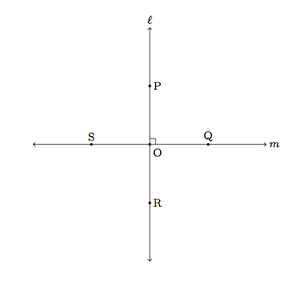# Defining Perpendicular Lines

Alignments to Content Standards: G-CO.A.1

## Task

Three students have proposed these ways to describe when two lines $\ell$ and $m$ are perpendicular:

1. $\ell$ and $m$ are perpendicular if they meet at one point and one of the angles at their point of intersection is a right angle.
2. $\ell$ and $m$ are perpendicular if they meet at one point and all four of the angles at their point of intersection are right angles.
3. $\ell$ and $m$ are perpendicular if they meet at one point and reflection about $\ell$ maps $m$ to itself.

Explain why each of these definitions is correct. What are some of the advantages and disadvantages with each?

## IM Commentary

The purpose of this task is to critically examine some different possible definitions of what it means for two lines to be perpendicular. All of these definitions are correct but they convey different information and build on different knowledge. Definition (a) is the simplest and most common and it is very closely related to (b). Though very similar, Definition (a) works from a weaker hypothesis than Definition (b) because the fact that the four angles made by perpendicular lines are right can be deduced, using supplementary and/or vertical angles, from the fact that one of angles is right. Since definitions should be as simple as possible, the weaker hypothesis of Definition (a) is preferable.

Definition (c) is very different in flavor from the first two. While it is mathematically correct, it is not the best definition because defining the reflection about $\ell$ requires knowing what it means for two lines to be perpendicular. Unless reflection about $\ell$ can be defined in some other way which does not use perpendicular lines, this leads to circular reasoning. Hence, although it is important to understand (c), this would not be a good choice for defining perpendicular lines. Nonetheless, reflections are fundamentally related to the notion of perpendicular lines and so it is very appropriate to consider the two together, understanding that perpendicular lines are a logical prerequisite to define reflections.

Work on finding the clearest, most economical definitions embodies MP6, Attend to Precision. Mathematical definitions need to be unambiguous and, ideally, they should convey the necessary information without unneeded hypotheses. Work on this task is ideally suited for class discussion as different students will likely react differently to the definitions and, through discussion, they will be able to come to a deeper understanding of what it means for lines to be perpendicular.

## Solution

1. Below is a picture of this situation which we will use for (a) and then (b). Here we have assumed that $\angle POQ$ is a right angle.The main advantage to this definition is its simplicity. It only makes an assumption about one of the four angles at $O$, as opposed to Definition (b) which assumes that all four are right angles. Also, unlike Definition (c) the only prior knowledge assumed is the definition of a right angle.

We also show here that Definition (a) implies Definition (b) (with fewer hypotheses). Note that angles $POQ$ and $QOR$ are supplementary since together they form $\ell$. Since $m(\angle POQ) = 90$ by hypothesis and $m(\angle POQ) + m(\angle QOR) = 180$ this means that $m(\angle QOR) = 90$, so it is also a right angle. The same reasoning can be applied to conclude that $m(\angle POS) = 90$ since, together with $\angle POQ$, this makes line $m$. Finally, for $\angle SOR$, it makes a vertical pair together with right angle $\angle POQ$ and so it is congruent to $\angle POQ$. Therefore $m(\angle SOR) = 90$. Thus all four angles made by $\ell$ and $m$ at $O$ are right angles.

2. This definition has the same prerequisites as (a), namely understanding of right angles. Unlike Definition (a), it assumes that all four angles made by $\ell$ and $m$ are right angles. Though this can be deduced as in the last paragraph above, this definition has the advantage of being natural: no one of the four angles is given special status as in the first definition. One disadvantage to this definition is that if we wish to show or deduce that a pair of lines $\ell$ and $m$ are perpendicular, we have four different angle computations to make instead of only one with the first definition.

3. This definition is accurate but is problematic because the usual definition of reflection about $\ell$ for a point $A$ not on $\ell$ is the following: reflection about $\ell$ maps $A$ to $B$ where $\ell$ is the perpendicular bisector of $\overline{AB}$. Thus the definition of reflection about $\ell$ assumes that we know what it means for line $\ell$ to be perpendicular to segment $\overline{AB}$. But this is the same as what it means for $\ell$ and $\overleftrightarrow{AB}$ to be perpendicular. In other words, reflections presume knowledge of what it means for two lines to be perpendicular. Therefore, reflections should not be used in the definition of perpendicular lines. It is important, however, for students to associate perpendicular lines to the concept of reflections even if reflections are not appropriate to define perpendicular lines.